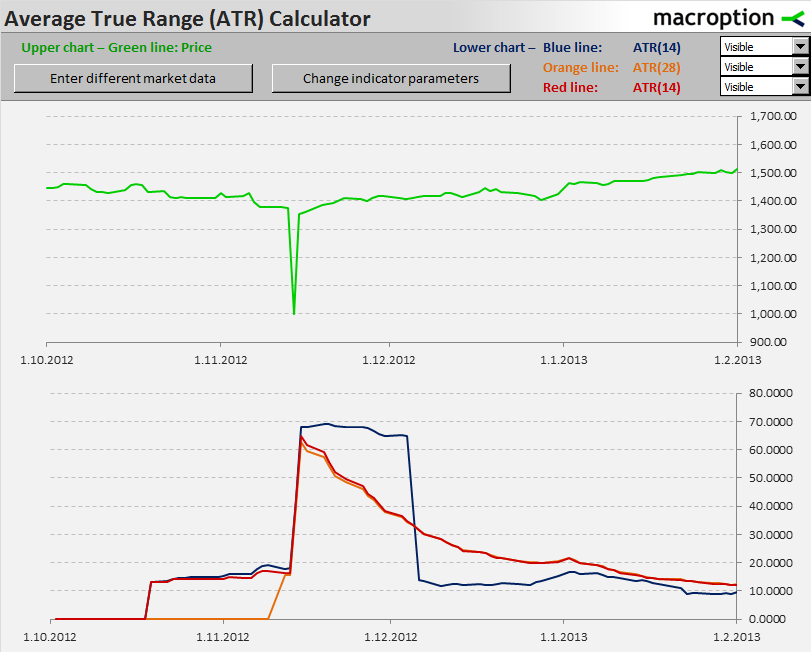# ATR Calculation Methods and Formulas

This page explains the calculation of Average True Range (ATR). We will look at all three commonly used calculation methods – simple, exponential, and the original Wilder's smoothing method. We will also compare the results of these methods on some examples, pointing out their different characteristics.

## Calculating True Range

Average True Range, as its name suggests, is the average of true range. If you want to understand and calculate ATR, you first need to understand and calculate true range, which is the greatest of these three:

• High minus low (like traditional range)
• High minus previous close
• Previous close minus low

So the formula for true range is: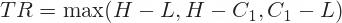... where H, L, C1 are high, low, and previous close, respectively.

For more detailed explanation of true range and some graphical examples, see True Range and How It Differs from Range.

## Calculating ATR from True Range

Once you have calculated true range for each bar, the next step is to calculate the average of these, which is the ATR that we want. There are several different methods for that and the three most common are the following:

• Simple Moving Average
• Exponential Moving Average
• J. Welles Wilder's Smoothing Method

### Simple Moving Average (SMA) Method

Simple moving average in the calculator) is mathematically arithmetic average – the sum of last n bars divided by n:... where:

• n is the ATR period length
• TRi is true range i bars ago

### Exponential Moving Average (EMA) Method

Exponential moving average puts greater weight on the most recent bars and smaller weight on older bars: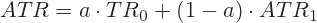... where:

• TR0 is true range for the current bar
• ATR1 is ATR calculated for the previous bar
• a is the smoothing factor, which is a function of the period length n: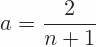The smoothing factor a is the weight of the current bar's true range and 1 – a is the weight of the previous bar's ATR. You always need the previous bar's ATR.

To calculate first ATR (when you don't have previous bar's ATR), just use the simple moving average method (arithmetic average of first n bars).

### Wilder's Smoothing Method

This is the method originally used for ATR calculation by ATR inventor J. Welles Wilder (explained in his book New Concepts in Technical Trading Systems, page 23). It has the same logic as exponential moving average (puts greater weight on the most recent bars), from which it only differs in the exact calculation of the smoothing factor: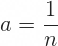For detailed guide to calculating all the three methods in Excel, see the ATR Excel tutorial.

## Which of the Methods is the Correct One?

In various resources you will find that one of the methods is the "right one" and the others are "incorrect". If you are looking for the "original" ATR, as presented by J. Welles Wilder, use Wilder's smoothing method.

However, there is no such thing as "correct" or "incorrect" calculation method and none of the methods is universally more profitable than the others when using ATR as part of the investment decision making process.

Of course, different methods lead to slightly different behaviour of ATR and may have strengths and weaknesses, depending on the particular purpose, trading style, and market conditions. But personally I find the selection of particular method less important than simply knowing what you are using and using it consistently. If you are familiar with trading strategies based on moving averages, it is very similar to the SMA vs. EMA (vs. another MA's) discussion. It is also similar to the discussion about the "best" period length.

## Comparing EMA/Wilder's vs. SMA ATR Calculation

Note: The EMA and Wilder methods are actually quite similar (you could already see that in the formulas above). There is in fact a direct relationship between period lengths of the two (2x-1), although it is not 100% accurate due to the data start problem explained below.

### Disadvantages of EMA/Wilder's ATR calculation method

One disadvantage is that both EMA and Wilder's method are more complicated to calculate, though this does not play any role if you have Excel or common technical analysis software. For some people it may also be less comfortable to think about and interpret.

A more serious issue is that the resulting ATR at a particular point of time can show different values for the same set of data depending on when you started to calculate ATR in the past. This is because EMA and Wilder's ATR calculation includes all data from start to the particular point of time (the data in distant past have very little weight in the final ATR value, but still do have some).

### Disadvantage of SMA ATR calculation method

One drawback of the simplified ATR calculation is that ATR sometimes changes due to a sharp True Range change N bars back, as the simple moving average window rolls, even when current True Range remains stable.

## ATR Calculation Methods Compared in One Chart

Below you can see the three ATR calculation methods in one chart. I have used S&P500 index daily data in 4Q2012, but I have changed the value on one day (to 1,000 on 14 November 2012) to create an extreme True Range value. You can see how ATR reacts differently to the spike in True Range under the three methods (blue = SMA, orange = EMA, red = Wilder, the period is 14 on all):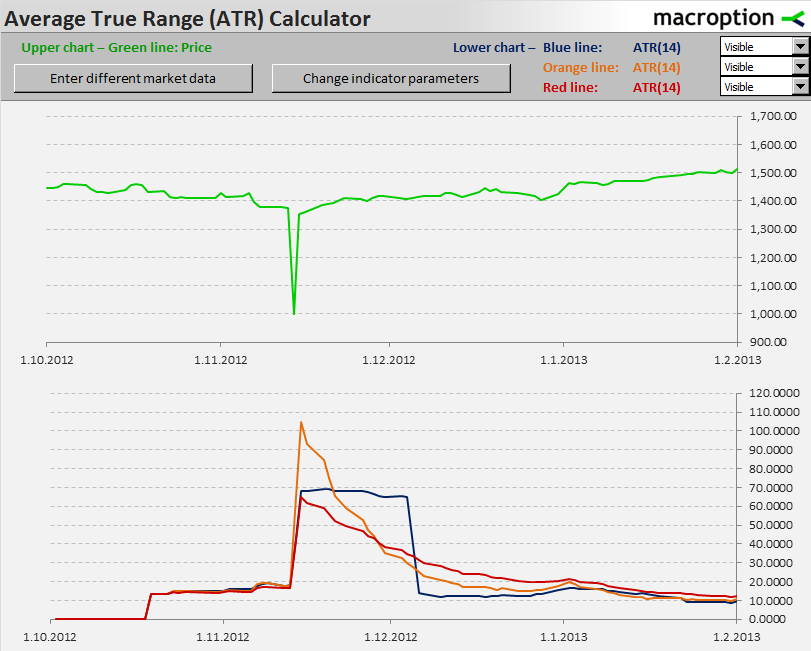As a result of the logic of individual calculation methods, the SMA ATR is elevated for 14 days (= ATR period) following the extreme price day, while the EMA and Wilder ATR start to gradually decline immediately after the True Range spike. However, EMA and Wilder ATR take longer time to return to the original level in the end. The difference is the same as the way simple and exponential moving averages react differently to price spikes, because the calculations are mathematically the same (you just use True Range instead of price on ATR).

Notice also the difference between EMA and Wilder ATR, which is a result of the difference in the smoothing factor. The numerator in the formula for a is 2 under EMA, but 1 under Wilder's method. Therefore, Wilder ATR with a particular period (n) is approximately the same as EMA ATR with double the period (2n, or 2n – 1 to be precise). The chart below shows the same as the one above, only the EMA ATR period was doubled to 28. You can see the EMA and Wilder ATR are almost the same now: